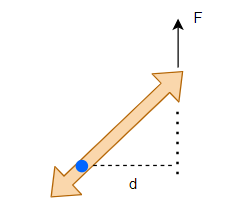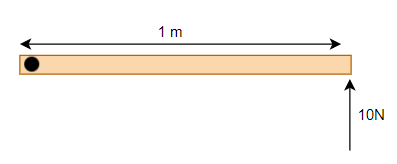# Torque Calculator

Note
• Enter the values of the two known variables in the text boxes
• Leave the text box empty for the variable you want to solve for
• Click on the calculate button.

Formula used
$\tau=Fd$

## What is Torque

This tendency of the force to rotate an object about some axis is called torque.It is the rotational counterpart of force. torque tends to rotate an body in the same way as force tends to change the state of motion of the body.Torque is given by
$\tau=Fd$
where
F-> Force Acting on the Body
d ->is the perpendicular distance from the pivot point to the line of action of force F
$\tau$ -> Torque
Torque is a vector quantity .It has both magnitude and direction. The SI unit of Force is Newton-m
It can be expressed in vector form as
$\mathbf{\tau} = \mathbf{F} \times \mathbf{r}$
Where
$\mathbf{F}$ is the force acting on the particle
$\mathbf{r}$ is the position vector of particle with respect to constant point
Torque can also be found using the below relation
$\tau = I \alpha$
Where
I ->Moment of Inertia
$\alpha$ -> Angular accleration
Another Relation which could be useful
$L= I \omega$
Where
L- > Angular Momentum
I -> Moment of Inertia
$\omega$ -> Angular Velocity

Example of Few questions where you can use this Torque Calculator
Question 1
A Force of 10N is acting on a rod hinge at one end as shown in below figureFind the torque acting
Solution
F = 10 N Now we can see from the figure force is acting perpendicular to the rod.So, d=1 m Now as per Torque formula
$\tau=Fd = 10 \times 1 = 10 \ N-m$

Question 2
The width of a door is 50 cm. If it is released by exerting a force of 1 N at its edge (away from the hinges).calculate the torque produced? Solution
F = 1 N, d=50 cm=.5 m
Now as per force formula
$\tau=Fd = 1 \times .5 = .5 \ N-m$

## How the Torque Calculator works

1. If force and distance are given, Torque is calculated as
$\tau= Fd$
2. If force and torque are given, distance is calculated as
$d= \frac {\tau}{F}$
3. If torque and distance are given, force is calculated as
$F = \frac {\tau}{d}$